EX T R E ME

AMAZING

DIGITIZED
DIFFERENTIAL
WINCH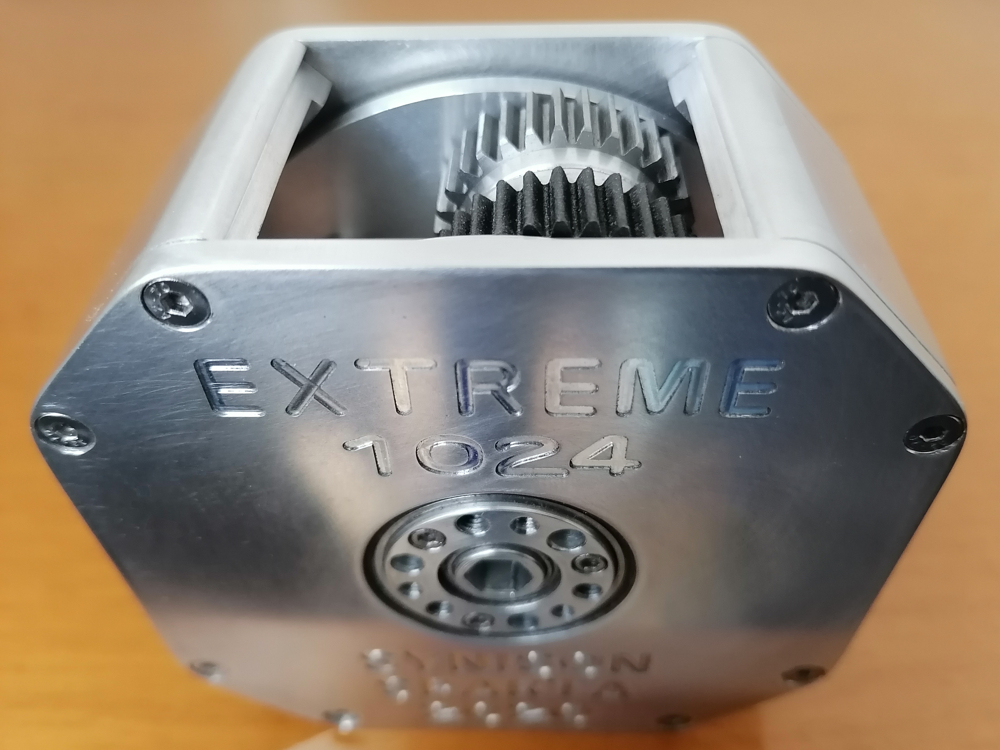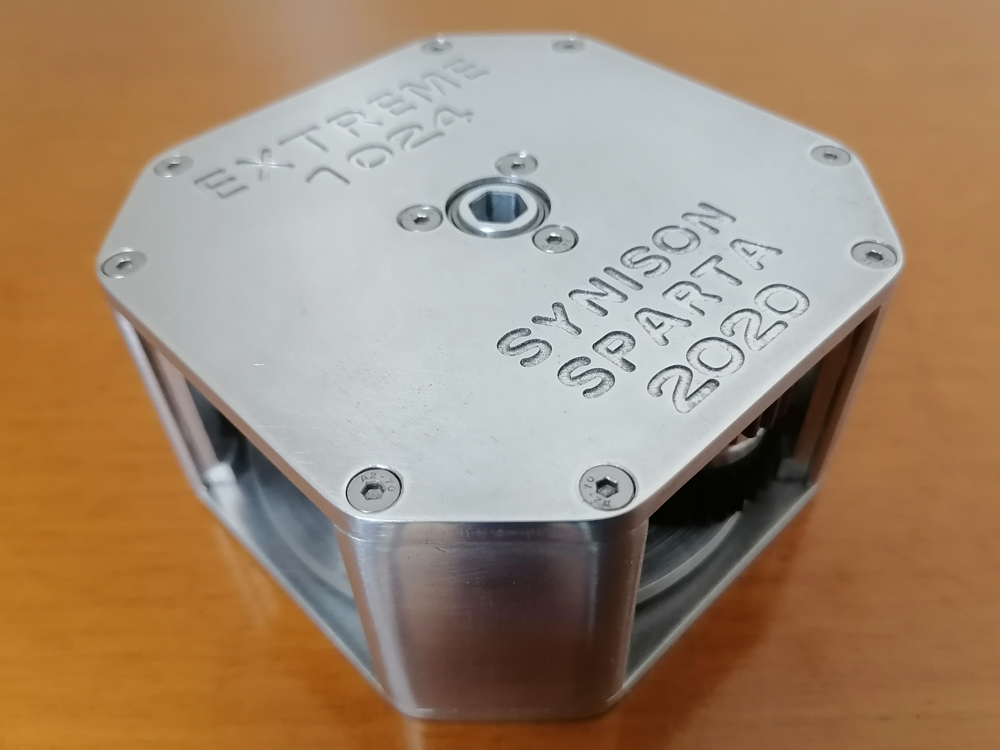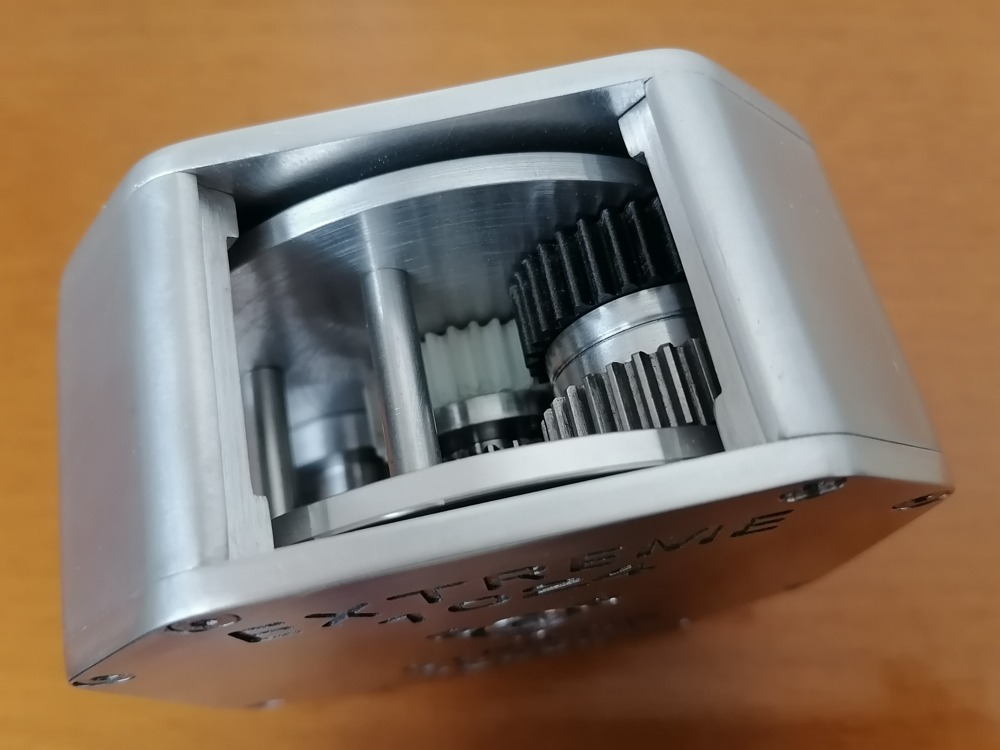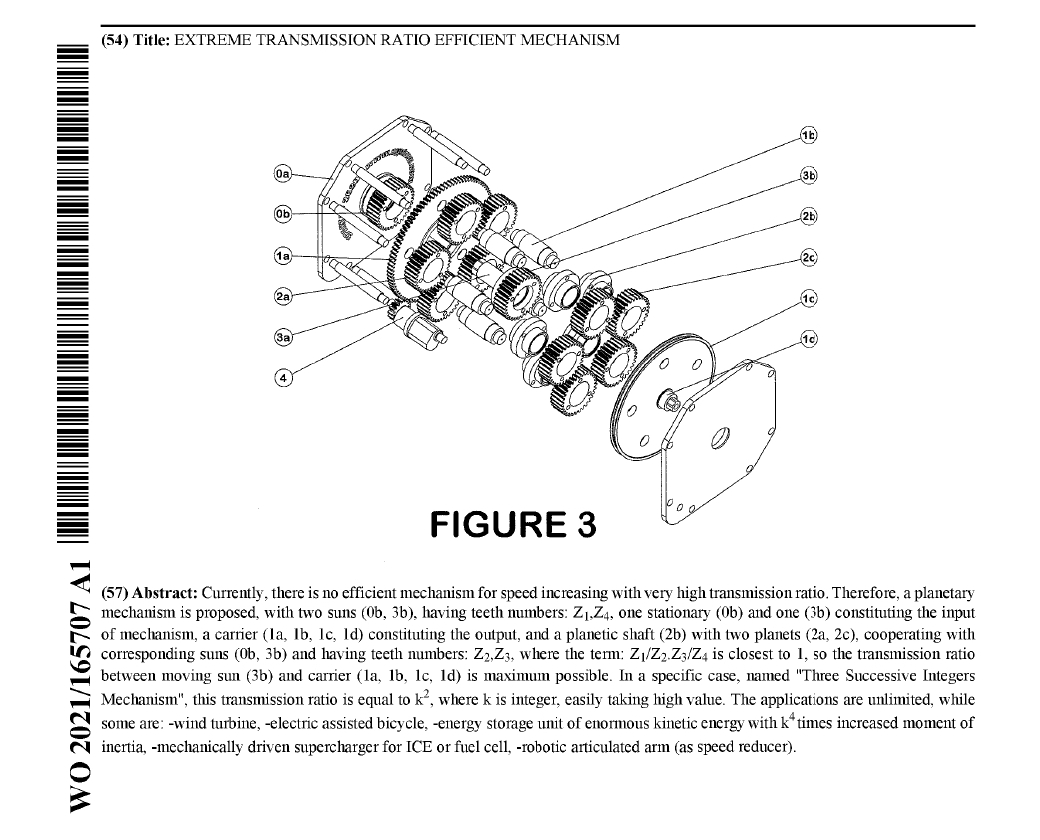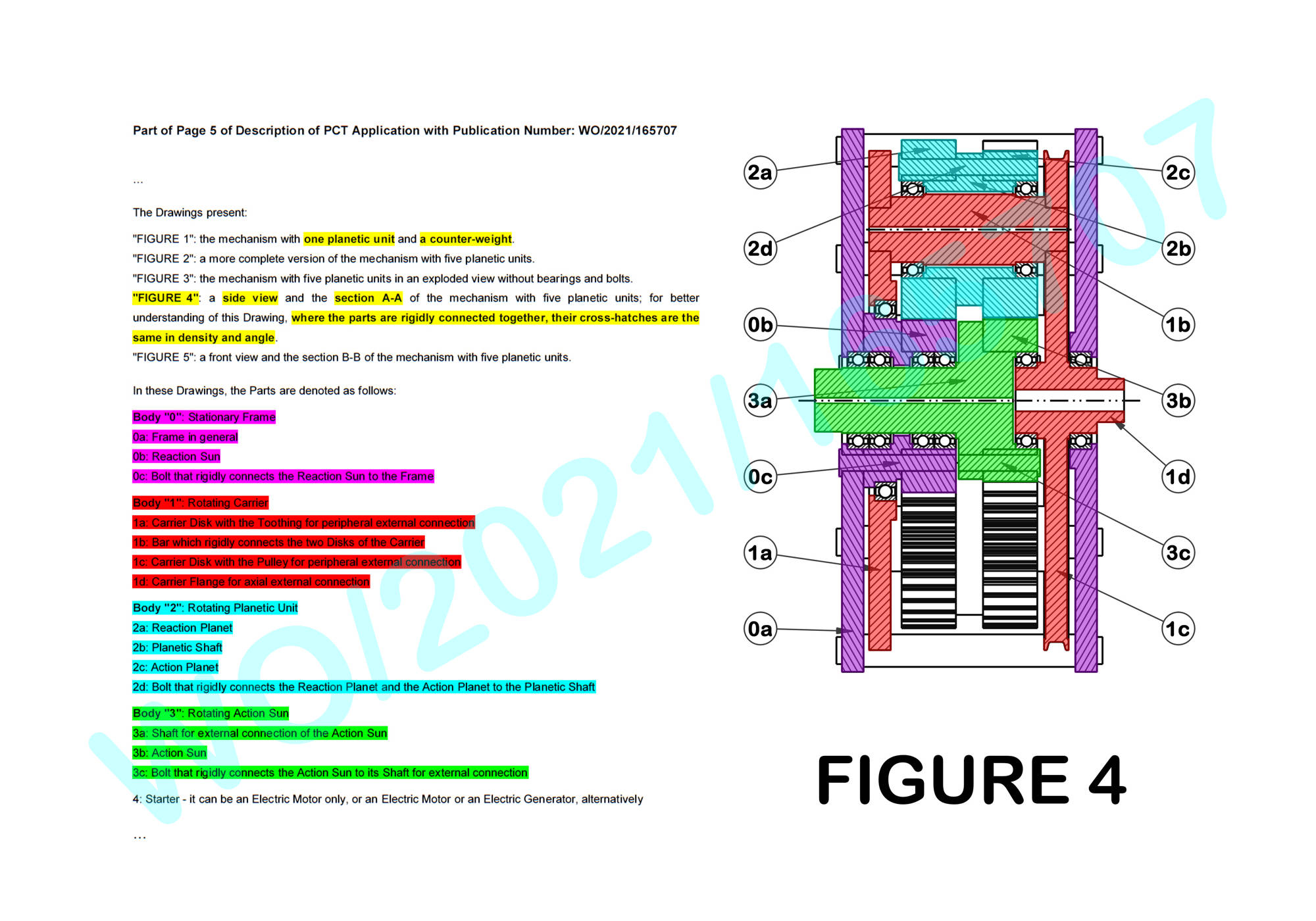The Differential Winch, being analogical, is the only purely mechanical mechanism that can achieve almost infinite transmission ratio in both directions: for speed reducing and speed increasing.
However, it has ONE only drawback: if it is based on friction then it has a limitation in output torque magnitude, and if not then either it has a limitation in output stroke, angular or linear, or it needs a rope or an untoothed belt with correspondingly infinite length.
The epic effort in the 19th century to solve this problem, by the digitalization of the Differential Winch, resulted in the Differential Pulley, achieving, however, a rather low value as its maximum transmission ratio.

The present Invention achieves a successful digitalization of this analogical Differential Winch, resulting in the simplest possible and the most efficient possible geared transmission mechanism with an extreme transmission ratio, named "EXTREME".
From another perspective, the present Invention is the child of the fertile marriage between the Differential Winch and the Three Successive Integers Conjecture.

Disclosure of the Invention:

The creation of the present Invention started as a development of the magic differential winch, but in a quite different way than its digitalization in the 19th century, which resulted in the Differential Pulley.

The main idea is the hybridization of the differential winch with the planetary mechanism, keeping the concept of the small difference between the diameters of the two working pulleys of the differential winch.

However, it is a paradox the fact that, although there is a great progress in every field of Mathematics and Mechanical Engineering, one, in order to be able to achieve the highest possible transmission ratio of a transmission mechanism, firstly has to overcome three deeply rooted perceptions that have been grown through the last decades, literally being "dogmas" without a reasonable basis.
Usually a planetary mechanism comprises internal gears and the perception that this is the best configuration is the first "dogma".
Acting in the opposite direction of this "dogma", a planetary mechanism with a rare configuration, using only external gears, has been formed.

This planetary mechanism, in general lines, has a frame, a gear, named "reaction sun", rigidly connected to the frame, the axis of which is named "central axis", with a teeth number Z1, a carrier supported on the frame and rotating about the central axis, a unit, named "planetic unit", supported on the carrier and rotating about an axis, named "planetic axis", at a distance from the central axis and parallel to it, and, finally, a gear, named "action sun", supported on the frame coaxially with the central axis and rotating about it, with a teeth number Z4.
The planetic unit consists of a shaft, named "planetic shaft", a gear, named "reaction planet", rigidly connected to the one end of the planetic shaft coaxially with the planetic axis and cooperating with the reaction sun, with a teeth number Z2, and a gear, named "action planet", rigidly connected to the other end of the planetic shaft coaxially with the planetic axis and cooperating with the action sun, with a teeth number Z3.

Although there are two pairs of cooperating gears, just because it is a planetary mechanism, it is considered that it is a single-stage one.
In order to make more clear the following analysis, each of these pairs is named "branch".

The relation between the speed of the carrier "ωc" and the speed of the action sun "ωa" is: ωc=ωa*1/(1-Z1/Z2*Z3/Z4), hence, the transmission ratio between the action sun and the carrier is: 1:1/(1-Z1/Z2*Z3/Z4), so, the closer the term: Z1/Z2*Z3/Z4 to 1, the higher the transmission ratio that is achieved. It is obvious that there is the need of small differences between the teeth numbers of the suns and the planets and, since in internal gearing there is the problem of interference of the teeth profiles when using teeth numbers with small difference and the involute profile, the use of only external gears -that means breaking the first "dogma"- is much more effective and this is a great advantage of the configuration of the planetary mechanism of the present invention, considering the transmission ratio level.

The second "dogma" is that the module of the teeth of the gears must be the same throughout the whole mechanism by a tacit admission, so there are very strict limits in teeth numbers setting, having to satisfy the "wrongly required" relation: Z1+Z2=Z4+Z3.
The power of this "dogma" has been amplified, even more, considering the cycloidal drive, since in internal gearing the relative equations are: Z1-Z2=1, and: Z4-Z3=1, thus the relation: Z1-Z2=Z4-Z3 is satisfied "automatically", without questioning for the equality of the modules of the two branches.

Trying to achieve the highest transmission ratio, one can set the partial ratio of the one branch to: Z1/Z2=(k-1)/k, where k is an integer, but then there is an immovable obstacle to set the other branch, satisfying the above "wrongly required" relation: Z1+Z2=Z4+Z3, so the next highest partial ratio of this branch is: Z1/Z2=(k-1)/(k+1), and then, always having to satisfy the above "wrongly required" relation: Z1+Z2=Z4+Z3, there are three options for the setting of the other branch:
a) Z3/Z4=(k+1)/(k-1), which results in: Z1/Z2*Z3/Z4=1, that is a useless situation since ωc is practically independent from ωa and action sun is always stationary as if it was welded on the frame,
b) Z3/Z4=(k-1)/(k+1),
c) Z3/Z4=k/k,
where just the third option renders the term: Z1/Z2*Z3/Z4=(k-1)/(k+1) most close to 1.
This result has already established a third "dogma", being stated as follows: when setting up the teeth number of each of the two branches of this planetary mechanism, the one branch must have always the partial ratio: 1:1 by a tacit admission, leaving the other branch to achieve an effective transmission ratio.

After the breaking of the second "dogma", accepting that there is one module for the one branch and another, different in general, module for the other branch, the present invention goes one more daring step forward, stating that the one, at least, module can be non-standard, thus the field of the options opens even wider.
So, if "L" is the distance between the central axis and the planetic axis, the new requirement is formed as:
2*L=(Z1+Z2)*moduleR=(Z4+Z3)*moduleA.
The one module, of course, can be a standard one and the other customized, in order to reduce the construction cost.

However, although the field of the options for the setting of the teeth numbers is rendered wider after the accepting of the differentiation of the two teeth modules, the third "dogma" remains still as an obstacle and leads only to the option: Z1/Z2=(k-1)/k and: Z3/Z4=k/k, while in this case the relation for the modules is: (2*k-1)*moduleR=2*k*moduleA, and this option nearly doubles, indeed, the transmission ratio, but that is all.

Fortunately, an earlier, purely mathematical work turned out to be the perfect assistance for the situation: in order to achieve the maximum possible transmission ratio, for this configuration, using a given mean of a set of teeth numbers, it is necessary to set the partial ratio of the second branch at a value competitive to the one of the first branch, an idea really revolutionary.

So, there is a mathematical proposal in the Number Theory, which can be named:
"Three Successive Integers Conjecture",
and can be stated as follows:
"For any given integer k, the combination of any four integers: n1, n2, n3, n4, between 2 and k+1, inclusive, for which the term: '1-n1/n2*n3/n4' is rendered non-zero minimum, is: n1=k-1, n2=k, n3=k+1, n4=k, therefore: 1-n1/n2*n3/n4=1/k2".

This prospective conjecture, being developed, numerically verified in a considerable range of integers and applied by the applicant of the present invention, is currently under an official examination for its novelty.

Therefore, the most efficient combination of teeth numbers is: Z1=k-1, Z2=k, Z3=k+1, Z4=k, which, according to the above, gives: ωc=ωa*k2, while its dyadic combination: Z1=k+1, Z2=k, Z3=k-1, Z4=k, gives exactly the same result, but also gives the smallest possible outer diameter of the mechanism, overall, and therefore this option will be exhaustively studied here as the most compact, but also as being representative for both cases.
Finally, the combination: Z1=k, Z2=k-1, Z3=k, Z4=k+1, and its dyadic: Z1=k, Z2=k+1, Z3=k, Z4=k-1, "approach 1 from above", that means that they produce the minimum possible term: Z1/Z2*Z3/Z4 which is at the same time greater than 1, and even more give a sign inversion: ωc=ωa*(1-k2).
The following relation applies for both of the first of the aforementioned combinations:
(2*k-1)*moduleR=(2*k+1)*moduleA,
while the following relation applies for both of their dyadic combinations:
(2*k+1)*moduleR=(2*k-1)*moduleA.

For a comparison, in prior state of the art, the usual setting of the teeth numbers, as described above, is: Z1=k-1, Z2=k+1, Z3=k, Z4=k, so that: Z1+Z2=Z4+Z3, resulting in a transmission ratio: 1:1/(1-(k-1)/(k+1)*k/k), so the ratio is: 1:(k+1)/2.
After the breaking of the second "dogma", but with the third "dogma" still remaining, this setting is rendered: Z1=k-1, Z2=k, Z3=k, Z4=k, resulting in a transmission ratio: 1:1/(1-(k-1)/k*k/k), so the ratio is: 1:k, that is almost the double of the previous result.
However, using the exactly same set of teeth numbers of the first of the above options (attention: not the same set of gears): k-1, k, k, k+1, but with a quite different arrangement, for the mechanism of the present invention we have:
Z1=k-1, Z2=k, Z3=k+1, Z4=k, resulting in transmission ratio:
1:1/(1-(k-1)/k*(k+1)/k), so the transmission ratio is: 1:k2, that is really a huge one.

Being more specific, for example if: k=20, then the transmission ratio for the arrangement of the prior state of the art is: 1:10.5, and after the breaking of the second "dogma" is: 1:20, while the transmission ratio of the present invention is: 1:400, a value undoubtedly without comparison.

With such a huge transmission ratio, with three only simple moving parts and the superiority of the involute, this mechanism, aiming to be the first single-stage efficient speed increaser with a very high transmission ratio, could be the sought one, the last decades, to achieve the required speed increasing in the field of the wind turbines, as well as in other application fields.

The present invention looks extremely simple; however there was a long-time hard struggle to beat the aforementioned three "dogmas", a struggle in the field of Mechanical Engineering and another in the field of Mathematics.
In fact, the effort to digitize the differential winch in a quite new way, as described above, very soon gave the formation of the just presented planetary mechanism, however with the other two "dogmas" still closely following it.
Since these "dogmas" collapsed, it was rather self-evident to apply the aforementioned prospective conjecture and design two non-standard gears with the standard involute but with a non-standard module.
So, the present invention could be a promising child of one more creative marriage of the Mathematics and the Mechanical Engineering.

Finally, looking at this issue from a wider perspective, it should be noted that the Extreme Transmission Ratio Efficient Mechanism, while radically differs (especially: in the present invention the amplitude of the involved oscillation is just zero) from the previous cases WO/2007/125373, WO/2009/040588, WO/2018/020279 and WO/2021/260400, falls within the broadly defined category under the name:
"DISTRIBUTIVE OSCILLATING TRANSMISSION" ("DOT").

M O R E (complete description, drawings, claims etc) as PDF

M O R E (complete description, drawings, claims etc) at WIPO

The most significant advantages are the incomparable simplicity of the whole mechanism and the use of the perfect INVOLUTE.
In its basic version, this mechanism includes only THREE moving parts, namely the action sun, the carrier and the planetic unit.
Moreover, its operation is based on the cooperation of only TWO pairs of cooperating gears.
As a result, the highest possible degree of efficiency is achieved for both operations, either to reduce or to increase the speed, and moreover this mechanism is rendered unique to be an efficient speed increaser.
All parts can be already existing components, except the two non-standard gears.
In fact, these two gears are standard ones with a scale factor: (2*k+1)/(2*k-1), or: (2*k-1)/(2*k+1), both scale factors being very close to 1, so for a medium level mass production the cost of them can be less than 110% of the cost of the standard gears; however, the cost of the whole mechanism according to the present invention can be easily less than the 50% of the cost of the whole mechanism according to the prior state of the art, for the same purposes and requirements.
So, this mechanism is characterized by easy construction, as well as easy and cost-effective operation and maintenance.

By just its design per se, this mechanism is limited in size, however with the addition of more planetic units and specifically with the use of up to five such planetic units, the load to be received by the involved teeth is ideally distributed to more simultaneously cooperating teeth pairs and thus achieves the minimum, allowed by the strength requirements, width of the toothings, with the best possible space exploitation.
Additionally, it is very important that, also by the design of this mechanism per se, the most balanced arrangement of the diameters of the gears is achieved, as a result of which the usual -and particularly problematic in relative cases, being also the weakest link in any power train- pinion is absolutely absent.
Regarding the application of this mechanism as a temporary -but even with a longer duration- energy storage unit, this proposal is superior to the classic flywheel, as for the same mass -therefore volume and weight- the apparent moment of inertia of the carrier as a whole -that is with all planetic units- is k4 times greater, with correspondingly huge margins of energy storage.
These advantages make this mechanism the ideal option for any case where an increasing of speed is required; however, in any case, also, where a drastic reduction of speed is required, this mechanism is superior to its existing competitors.

In conclusion, these are a number of merits which are easily contrasted as advantages over a competition, which, in fact, is rather moderate.

M O R E (complete description, drawings, claims etc) as PDF

M O R E (complete description, drawings, claims etc) at WIPO

Since this Work refers persistently to the Number Theory and the Publication Number of it is WO/2021/165707, discover what the Number 1 6 5 7 0 7 is!